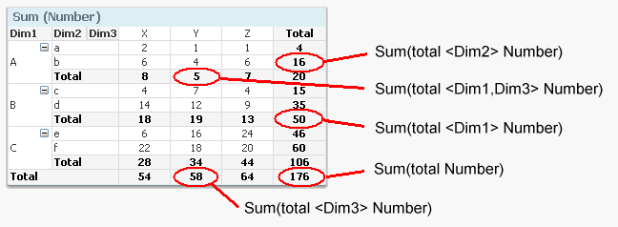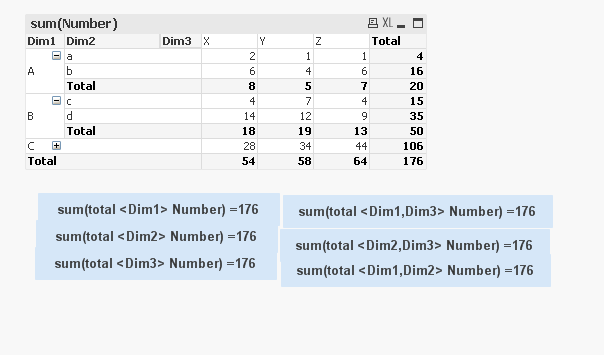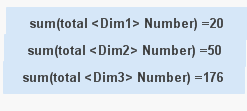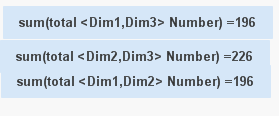# QlikView App Dev

Discussion Board for collaboration related to QlikView App Development.

Announcements
Join this live chat April 6, 10AM EST - QlikView to Qlik Sense REGISTER
cancel
Showing results for
Search instead for
Did you mean:Not applicable

## Aggr Expression is not working

Hi All,

I am replicating HIC blog example given in When should the Aggr() function NOT be used?

hic

stalwar1

swuehl

jagan

Manish Kachhia

Given Example:Replication with the expressions:But for me I am getting 176 values for all the expressions.

I want to replicate the same. How to write the expressions?

Thanks in advance.

Data:

 Dim1 Dim2 Dim3 Number A a X 2 A b X 6 A a Y 1 A b Y 4 A a Z 1 A b Z 6 B c X 4 B d X 14 B c Y 7 B d Y 12 B c Z 4 B d Z 9 C e X 6 C f X 22 C e Y 16 C f Y 18 C e Z 24 C f Z 20
4 RepliesMVP

Is this you are expecting?Before develop something, think If placed (The Right information | To the right people | At the Right time | In the Right place | With the Right context)Not applicable
Author

I am expecting this:MVP

I assume, these values you need from KPI(Text Object)

FYI, PFAIf you don't have license, Follow those pattern

16 =Sum(TOTAL {<Dim2={'b'}>} Number)

5 =Sum(TOTAL {<Dim3={'Y'}, Dim1={'A'}>} Number)

50 = Sum(TOTAL {<Dim1={'B'}>} Number)

58 =Sum(TOTAL {<Dim3={'Y'}>} Number)

176 =Sum(TOTAL Number)

Before develop something, think If placed (The Right information | To the right people | At the Right time | In the Right place | With the Right context)Champion II

I think you have misunderstood the usage of TOTAL here, It  needs to be used in a chart where you have many dimensions and only want the TOTALs referring to a Select dimension and ignoring other Dimensions,, try the total keyword in a straight table with and see for yourself.

in the Text box there are no dimensions to aggregate on, just using the set analysis is enough, the total keyword here is irrelevant, so in text boxes just use

16 =Sum({<Dim2={'b'}>} Number)

5 =Sum({<Dim3={'Y'}, Dim1={'A'}>} Number)

50 = Sum({<Dim1={'B'}>} Number)

58 =Sum({<Dim3={'Y'}>} Number)

176 =Sum(Number)